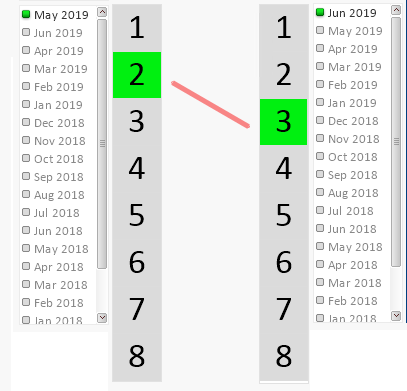# QlikView App Development

Discussion Board for collaboration related to QlikView App Development.

Announcements
Leverage your QlikView investment to modernize BI – see how! Join Group
cancel
Showing results for
Did you mean:
HighlightedContributor III

## Set analysis AND combination

I want to count unique DIMENSION1 where there is a record that has DIMENSION2 = 'VAL 1' AND DIMENSION3 = 'VAL A' but also has a record DIMENSION2 = 'VAL 2' AND DIMENSION3 = 'VAL B'.

Now normally I would attempt this in the script but in this case, I need to look at the situation dynamically (so the user can pick which value combinations are relevant).

Is this possible in set analysis?

Tags (1)
1 Solution

Accepted Solutions
HighlightedMVP

## Re: Set analysis AND combination

Try this

``=Count({\$<DIM1 = P({<DIM2 = {'C'}, DIM3 = {'CAT5'}>})*P({<DIM2 ={'A'}, DIM3 = {'CAT1'}>})>} DISTINCT DIM1)``
6 Replies
HighlightedCreator III

## Re: Set analysis AND combination

Hey Felix,
In your demo you want to count the number of YES by the selection of the user?
So if i choose
DIM1 = 1
DIM2 = A
DIM3 = CAT1

The expression should return 1?
HighlightedSpecialist II

## Re: Set analysis AND combination

Count({\$<DIMENSION2 = {'VAL 1'}, DIMENSION3 = {'VAL A'} > + < DIMENSION2 ={'VAL 2'} ,DIMENSION3 ={'VAL B'}>} DIMENSION1)

HighlightedCreator III

## Re: Set analysis AND combination

if you want to count the YES depending on the user's selection try this one:
=COUNT({<DIM1={'\$(=GetFieldSelections(DIM1))'} ,DIM2={'\$(=GetFieldSelections(DIM2))'} , DIM3={'\$(=GetFieldSelections(DIM3))'} >} IF(COUNTME='YES', COUNTME))
HighlightedMVP

## Re: Set analysis AND combination

Try this

``=Count({\$<DIM1 = P({<DIM2 = {'C'}, DIM3 = {'CAT5'}>})*P({<DIM2 ={'A'}, DIM3 = {'CAT1'}>})>} DISTINCT DIM1)``
HighlightedContributor III

## Re: Set analysis AND combination

Hi Gf,
Sorry my ability to articulate the problem isn't great and I believe that what you have set out here would work if I had the ability to do the COUNTME field but I only included that in the demo file to show the relevant lines. I can't do this in the real example.

Some context might help - we're looking at a bunch of students that have achieved grades A-E by months. I'm trying to create a visualisation that shows how many students that were achieving grade Bs in May 2019 and getting As in Jun 2019 based on selection:HighlightedContributor III

## Re: Set analysis AND combination

This seems to work.. That is some fantastic set analysis witchcraft. I'll look up the P() and what that is up to.

Thank you sir.